# Mathematical Modelling for Natural Convective Heat Transfer of Nanofluid inside a Prismatic Enclosure with Various Thermal Boundary Conditions

Mathematical Modelling for Natural Convective Heat Transfer of Nanofluid inside a Prismatic Enclosure with Various Thermal Boundary Conditions

M.S. Alam

Department of Mathematics, Jagannath University, Dhaka-1100, Bangladesh

Department of Mathematics & Statistics, College of Science, Sultan Qaboos University, P. O. Box 36, Postal Code-123 Al-Khod, Muscat, Sultanate of Oman

Corresponding Author Email:
msalam631@yahoo.com
Page:
162-170
|
DOI:
https://doi.org/10.18280/mmep.030402
N/A
|
Accepted:
N/A
|
Published:
31 December 2016
| Citation

OPEN ACCESS

Abstract:

In this paper, the problem of unsteady natural convective heat transfer of copper-water nanofluid inside a prismatic enclosure is studied numerically. The top inclined walls are considered at constant low temperature, two vertical walls are adiabatic whereas the bottom wall of the enclosure is heated isothermally as well as non-isothermally. The governing non-linear partial differential equations have been solved by using Galerkin weighted residual based finite element method. Comparison with previously published work is performed and excellent agreement between the results is observed. Numerical results have been presented graphically in the form of streamlines, isotherms and average Nusselt number for various model parametrs such as Rayleigh number and solid volume fraction of nanoparticles with two different thermal boundary conditions. The obtained numerical results indicate that the rate of heat transfer is  higher for uniformly heat bottom wall as compared to non-uniformly heat bottom wall.

Keywords:

modelling, nanofluid, natural convection, prismatic enclosure, finite element method

1. Introduction

Nanotechnology plays an important role for the development of 21st century modern micro- and nano-devices. Recent advances in nanotechnology have allowed researchers to study the next generation heat transfer nanofluids, which consist of a base fluid containing a suspension of ultra-fine nanometer-sized (usually less than 100 nm) solid particles. Nanoparticles can be metal particles such as Cu, Fe, Ag or Au or metallic oxides or nonmetallic oxide particles - CuO, Al2O3, TiO2, SiO2 etc. and the host fluid can be water, ethylene glycol, engine oils etc. Nanofluids is the term coined by Choi  to describe this new class of nanotechnology based heat transfer fluid that exhibits thermal properties superior to their host fluids or of conventional particle fluid suspensions. Significant features of nanofluids over base fluids include enhanced thermal conductivity, greater viscosity, and enhanced value of critical heat flux. Compared to the existing techniques for enhancing heat transfer, the nanofluids show a superior potential for increasing heat transfer rates in various cases. Choi  reported the possible use of nanofluids in a wide variety of industries ranging from transportation, energy production and supply to electronics, textiles and paper production. Recently, Uddin et al.  studied fundamental aspects of nanofluids with their evolution, applications and new theorem. Numerous researchers have studied and reported results on convective heat transfer in nanofluids considering various flow and thermal conditions in different geometries but less attention has been given on the prismatic shape enclosure, though this type of enclosure has many engineering applications such as solar energy collectors and conventional attic spaces of greenhouses and buildings with pitched roofs. Recently, Salma et al.  studied the free convective flow and heat transfer characteristics of alumina-water nanofluid inside a prismatic enclusure with sinosoidal temperature distribution on the bottom wall. Therefore, the objective of this work is to analyze the heat transfer characteristics of copper-water nanofluid inside a  prismatic enclosure with uniform and non-uniform heated bottom wall.

2. Physical and Mathematical Modelling

Let us consider an unsteady two-dimensional viscous, incompressible laminar natural convection flow of copper-water nanofluid inside a prismatic enclosure: See Figure 1 for schematic diagram and geometrical details. The horizontal bottom wall is heated isothermally as well as non-isothermally while the inclined walls are maintained at a constant lower temperature ${{T}_{c}},$ and the vertical walls are insulated. It is also assumed that thermal equilibrium exists between the base fluid and nanoparticles, and no slip occurs between the two media. The physical properties of the nanofluid are considered to be constant except the density variation in the body force term of the momentum equation, which is estimated by the Boussinesq approximation. The gravitational acceleration acts in the negative $y-$direction. All solid boundaries are assumed to be rigid no-slip walls. Then under these assumptions, the governing equations for unsteady natural convection flow can be written as:

$\frac{\partial u}{\partial x}+\frac{\partial v}{\partial y}=0$                                   (1)

${{\rho }_{nf}}(\frac{\partial u}{\partial t}+u\frac{\partial u}{\partial x}+v\frac{\partial u}{\partial y})=-\frac{\partial p}{\partial x}+{{\mu }_{nf}}(\frac{{{\partial }^{2}}u}{\partial {{x}^{2}}}+\frac{{{\partial }^{2}}u}{\partial {{y}^{2}}})$                      (2)

\begin{align} & {{\rho }_{nf}}(\frac{\partial v}{\partial t}+u\frac{\partial v}{\partial x}+v\frac{\partial v}{\partial y})=-\frac{\partial p}{\partial y}+{{\mu }_{nf}}(\frac{{{\partial }^{2}}v}{\partial {{x}^{2}}}+\frac{{{\partial }^{2}}v}{\partial {{y}^{2}}}) \\ & +{{(\rho \beta )}_{nf}}g(T-{{T}_{c}}) \\ \end{align}                       (3)

${{(\rho {{c}_{p}})}_{nf}}(\frac{\partial T}{\partial t}+u\frac{\partial T}{\partial x}+v\frac{\partial T}{\partial y})={{k}_{nf}}(\frac{{{\partial }^{2}}T}{\partial {{x}^{2}}}+\frac{{{\partial }^{2}}T}{\partial {{y}^{2}}})$                        (4)

## 1.png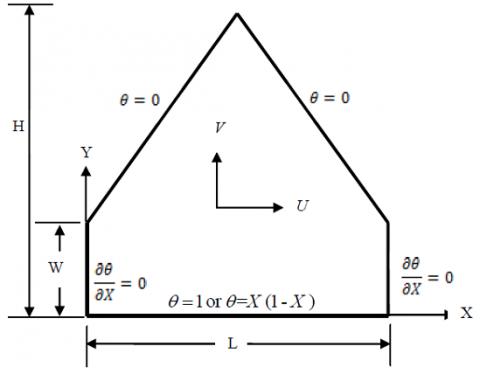Figure 1.  Schematic diagram of the physical model

3. Thermal and Physical Properties of Nanofluid

Thermal and physical properties of nanofluids are the properties which are important to enhance the thermal performance of nanofluids. These are viscosity, density, thermal diffusivity, heat capacitance, thermal conductivity and thermal expansion coefficient. Thermal performance of an engineering equipment mainly depends on how thermal properties behave in varied operating conditions. Operating parameters are variation in temperature, ambient conditions, type of base fluid, particle size, and shape of the nanoparticles and volume concentration. Considering all these parameters, selection of appropriate nanofluid is necessary for optimum performance. The following formulas have been used to compute the thermal and physical properties of the nanofluids under considerations:

The effective viscosity of the nanofluid may be expressed in the following form

${{\mu }_{nf}}={{\mu }_{bf}}{{(1-\phi )}^{-2.5}}$                            (5)

where $\phi$ is nanoparticles volume fraction.

The effective density of nanofluid is given as

${{\rho }_{nf}}=(1-\phi ){{\rho }_{bf}}+\phi {{\rho }_{sp}}$                                (6)

The thermal diffusivity of the nanofluid is given by

${{\alpha }_{nf}}=\frac{{{k}_{nf}}}{{{(\rho {{c}_{p}})}_{nf}}}$                        (7)

The heat capacitance of the nanofluid is given by

${{(\rho {{c}_{p}})}_{nf}}=(1-\phi ){{(\rho {{c}_{p}})}_{bf}}+\phi {{(\rho {{c}_{p}})}_{sp}}$                 (8)

The thermal conductivity of nanofluid is given by

$\frac{{{k}_{nf}}}{{{k}_{bf}}}=\frac{{{k}_{sp}}+2{{k}_{bf}}-2({{k}_{bf}}-{{k}_{sp}})\phi }{{{k}_{sp}}+2{{k}_{bf}}+({{k}_{bf}}-{{k}_{sp}})\phi }$                 (9)

The thermal expansion coefficient is given by

${{(\rho \beta )}_{nf}}=(1-\phi ){{(\rho \beta )}_{bf}}+\phi {{(\rho \beta )}_{sp}}$                                            (10)

$\text{Cu}$-water nanofluid has been studied for the present work, and this type of nanofluid is highly used in industries due to its improved thermophysical properties availability, and economic considerations. The thermophysical properties of pure water (${{\text{H}}_{\text{2}}}\text{O}$) and copper$\text{(Cu)}$ are presented in Table 1.

Table 1. Thermophysical properties of pure water and solid nanoparticles

 Physical properties water $\left( {{\text{H}}_{\text{2}}}\text{O} \right)$ $\text{Cu}$ ${{\text{c}}_{\text{p}}}\left[ \text{J/kgK} \right]$ 4179 385 $\text{ }\!\!\rho\!\!\text{ }\left[ \text{kg/}{{\text{m}}^{\text{3}}} \right]$ 997.1 8933 $\text{k}\left[ \text{W/mK} \right]$ 0.613 400 $\text{ }\!\!\mu\!\!\text{ }\left[ \text{Ns/}{{\text{m}}^{\text{2}}} \right]$ 0.001003 - $\text{ }\!\!\beta\!\!\text{ }\!\!\times\!\!\text{ 1}{{\text{0}}^{\text{-5}}}\left[ \text{1/K} \right]$ 21 1.67 $\alpha \text{ }\!\!\times\!\!\text{ 1}{{\text{0}}^{\text{-7}}}\left[ {{\text{m}}^{\text{2}}}\text{/s} \right]$ 1.47 1163.1 $\Pr$ 6.8377 -
4. Initial and Boundary Conditions

The initial and boundary conditions for the above stated model are as follows:

For $t=0;$ $u=v=0,$$T=0,$$p=0$                                         (11a)

For $t>0;$

at the bottom wall: $T={{T}_{h}}\,\,or\,\,T={{T}_{c}}+\left( {{T}_{h}}-{{T}_{c}} \right)\left( \frac{x}{L} \right)\left( 1-\frac{x}{L} \right)$ (11b)

at the top inclined walls: $T={{T}_{c}}$                                       (11c)

at the vertical walls: $\frac{\partial T}{\partial x}=0$                                            (11d)

at all solid boundaries:$u=v=0$                                       (11e)

where the variables and the related quantities are defined in the nomenclature.

5. Dimensional Analysis

Dimensional analysis is one of the most important mathematical tools in  the study of  fluid mechanics. To describe several transport mechanisms in nanofluids, it is meaningful to make the conservation equations into non-dimensional form. The advantages of non-dimensionalization are as follows: (i) non-dimensionalization gives freedom to analysis for any system irrespective of their material properties. (ii) one can easily understand the controlling flow parameters of the system, (iii) make a generalization of the size and shape of the geometry, and (iv) before doing experiment one can get insight of the physical problem. These aims can be achieved through the appropriate choice of scales.Therefore in order to obtain the dimensionless form of the governing equations (1)-(4) together with the boundary conditions (11) we introduce the following non-dimensional variables:

\left. \begin{align} & X=\frac{x}{L},\ Y=\frac{y}{L},\ U=\frac{u}{({{\alpha }_{bf}}/L)},\ V=\frac{v}{({{\alpha }_{bf}}/L)},\ \tau =\frac{t{{\alpha }_{bf}}}{{{L}^{2}}},\ \\ & P=\frac{p{{L}^{2}}}{{{\rho }_{bf}}\alpha _{bf}^{2}},\ \theta =\frac{T-{{T}_{c}}}{{{T}_{h}}-{{T}_{c}}},\Pr =\frac{{{\upsilon }_{bf}}}{{{\alpha }_{bf}}},Ra=\frac{g{{\beta }_{bf}}\left( {{T}_{h}}-{{T}_{c}} \right){{L}^{3}}}{{{\upsilon }_{bf}}{{\alpha }_{bf}}} \\ \end{align} \right\}              (12)

Now employing the relation (12) into equation (1)-(4), we obtain the following nonlinear differential equations:

$\frac{\partial U}{\partial X}+\frac{\partial V}{\partial Y}=0$                               (13)

$\frac{\partial U}{\partial \tau }+U\frac{\partial U}{\partial X}+V\frac{\partial U}{\partial Y}=-(\frac{{{\rho }_{bf}}}{{{\rho }_{nf}}})\frac{\partial P}{\partial X}+(\frac{{{\upsilon }_{nf}}}{{{\upsilon }_{bf}}})\Pr (\frac{{{\partial }^{2}}U}{\partial {{X}^{2}}}+\frac{{{\partial }^{2}}U}{\partial {{Y}^{2}}})$             (14)

\begin{align} & \frac{\partial V}{\partial \tau }+U\frac{\partial V}{\partial X}+V\frac{\partial V}{\partial Y}=-(\frac{{{\rho }_{bf}}}{{{\rho }_{nf}}})\frac{\partial P}{\partial Y}+(\frac{{{\upsilon }_{nf}}}{{{\upsilon }_{bf}}})\Pr (\frac{{{\partial }^{2}}V}{\partial {{X}^{2}}}+\frac{{{\partial }^{2}}V}{\partial {{Y}^{2}}}) \\ & +Ra\Pr \frac{{{(\rho \beta )}_{nf}}}{{{\beta }_{bf}}{{\rho }_{nf}}}\theta \\ \end{align}           (15)

$\frac{\partial \theta }{\partial \tau }+U\frac{\partial \theta }{\partial X}+V\frac{\partial \theta }{\partial Y}=(\frac{{{\alpha }_{nf}}}{{{\alpha }_{bf}}})(\frac{{{\partial }^{2}}\theta }{\partial {{X}^{2}}}+\frac{{{\partial }^{2}}\theta }{\partial {{Y}^{2}}})$                                 (16)

The non-dimensional inital and boundary conditions can also be written as follows:

For $\tau =0;$$U=V=0,$$\theta =0$, $P=0$                   (17a)

For $\tau >0;$

at the bottom wall : $\theta =1\,\,or\,\,\theta =X\left( 1-X \right)$                         (17b)

at the top inclined walls: $\theta =0$                              (17c)

at the vertical walls: $\frac{\partial \theta }{\partial X}=0$                                (17d)

at all solid boundaries:$U=V=0$              (17e)

6. Average Nusselt Number

The parameter of the engineering interest for the present problem is the average Nusselt number ($Nu$) along the bottom heated wall which is calculated from the following expression

$Nu=-\frac{{{k}_{nf}}}{{{k}_{bf}}}\int\limits_{0}^{1}{\frac{\partial \theta }{\partial Y}}dX$                   (18)

7. Computational Procedure

The governing dimensionless equations (13)-(16) along with the boundary conditions (17) have been solved numerically by employing Galerkin weighted residual based finite element method. The details of this method is well described by Zienkiewicz and Taylor , and Reddy and Gartling . In this method, the solution domain is discretized into finite element meshes, which are composed of non-uniform triangular elements.  The six node triangular elements are used in this work for the development of the finite element equations. All six nodes are associated with velocities as well as  temperature; only the corner nodes are associated with pressure. This means that a lower order polynomial is chosen for pressure and which is satisfied through the continuity equation. Then the nonlinear governing partial differential equations (i. e. conservation of mass, momentum and energy equations) are transferred into a system of integral equations by applying Galerkin weighted residual method. The integration involved in each term of these equations is performed by using Gauss's quadrature method. The nonlinear algebraic equations so obtained are modified by imposition of boundary conditions. To solve the set of the global nonlinear algebraic equations in the form of matrix, the Newton-Raphson iteration technique has been adapted through partial differential equation solver with MATLAB interface. The convergence criterion of the numerical solution along with error estimation has been set to $\left| {{\Phi }^{m+1}}-{{\Phi }^{m}} \right|\le {{10}^{-5}}$ , where $\Phi$is the general dependent variable $(U,\ V,\ \theta )$ and $m$is the number of iteration.

8. Code Validation

The computational model is validated against the problem of laminar natural convection in an isosceles triangular enclosure for clear fluid studied by Holtzman et al. . The cavity was heated isothermally from below and symmetrically cooled from above. The comparison of the average Nusselt number (at the hot bottom surface) between the result of the present code and the results found in the literature (Holtzman et al. ) for different aspect ratios, AR are documented in Table 2. The comparisons reveal an excellent agreement with the reported studies. This validation boosts the confidence in the numerical outcome of the present study.

Table 2. Comparison of average Nusselt number $Nu$ with those of Holtzman et al.  for $Ra={{10}^{5}}$, $\Pr =0.70$ and $\phi =0$in the present model.

 AR Holtzman et al.  Present results 1.0 1.80 1.82 0.5 2.19 2.20 0.2 2.48 2.50
9. Mesh Generation

In the finite element method, the mesh generation is the technique to subdivide a domain into a set of sub-domains, called finite element, control volume, etc. The discrete locations are defined by the numerical grid, at which the variables are to be calculated. It is basically a discrete representation of the geometric domain on which the problem is to be solved. Meshing the complicated geometry make the finite element method a powerful technique to solve the boundary value problems occurring in a range of engineering applications . Fig. 2 displays mesh configuration of the present physical domain with triangular finite elements.

## 2.pngFigure 2. Mesh generation of the prismatic shape cavity

10. Results and Discussion

The numerical computation has been carried out through the finite element method in order to analyze the unsteady natural convective heat transfer and fluid flow of copper-water nanofluid inside a prismatic enclosure with different thermal boundary conditions on the bottom heated wall. Thus in this section, the obtained numerical results have been displayed graphically in the form of streamlines and isotherms for various values of the Rayleigh number $\left( Ra \right)$and solid volume fraction of the nanoparticles $\left( \phi \right)$. In addition, the rate of  heat transfer in terms of the average Nusselt number at the bottom heated wall is also calculated and displayed in graphically for the above mentioned parameters with two differnt cases.

10.1 Effects of Rayleigh number$\left( Ra \right)$ on streamlines

The effects of Rayleigh number $\left( Ra \right)$on the streamlines for two different cases have been shown in Fig. 3.  It is seen from this figure that the trend of streamlines are similar for all cases. There are two symmetric circulation cells formed inside the enclosure. With the increasing value of Rayleigh number $\left( Ra \right)$,  the streamlines are less dense near the central vertical line. It is noteworthy to mention that the central cores of the circulatory cells increase in size for higher values of Ra indicate the greater strength of the flow. Higher flow intensity is seen for the base fluid in comparison to the nanofluid.

10.2  Effects of Rayleigh number$\left( Ra \right)$ on isotherms

The influence of Rayleigh number (Ra) on isotherms for the present configuration has been demonstrated in Fig. 4 for both cases. The pattern of isotherms is smooth for low Ra and is symmetric to the central vertical line for all the considered values of Ra. At higher Rayleigh number, a thermal plume rises from the middle of the bottom wall because of the thermal boundary conditions. The isothermal lines for pure water are more distorted than that of nanofluid.

10.3 Effects of nanoparticles volume fraction$\left( \phi \right)$ on streamlines

Streamlines corresponding to different solid volume fraction $\left( \phi \right)$of the nanoparticles are shown in Fig. 5 for both cases. It is seen from the figure that the trend of streamlines are similar for all cases. There are two symmetric circulation cells formed inside the enclosure. With the increasing value of $\left( \phi \right)$ the streamlines are less dense near the central vertical line. It is noteworthy to mention that the central core of the circulatory cell is reduced in size for higher nanoparticle concentration. Higher flow intensity is seen for the base fluid.

10.4 Effects of nanoparticles volume fraction$\left( \phi \right)$ on isotherms

The influence of nanoparticle volume fraction on isotherms for the present configuration has been demonstrated in Fig. 6 for both cases. The pattern of isotherms are smooth and are symmetric to the central vertical line for all the considered values of $\left( \phi \right)$. A thermal plume rises from the middle of the bottom wall because of sinusoidal boundary temperature. At $\phi =0,$, that is in the case of base fluid, the higher temperature lines remain near the hot bottom wall. These lines move toward the cold top inclined walls due to the increasing values of $\left( \phi \right)$. This happens due to the presence of nanoparticle in the fluid and buoyancy effect which causes higher temperature gradient and thus the isothermal lines move from hot walls to the cold wall.

10.5 Average Nusselt number

Average Nusselt number for different values of Rayleigh number $\left( Ra \right)$and nanoparticles volume fraction $\left( \phi \right)$for both uniform and non-uniform heating bottom wall have shown in Fig. 7(a), and Fig. (b) respectively. General observation is that the average Nusselt number increases with the increasing values of Rayleigh number as well as  nanoparticles volume fraction and it is utilized to represent the overall heat transfer rate within the domain. But thehighest heat transfer rate is observed for uniformly bottom heated wall case.

## 3a.png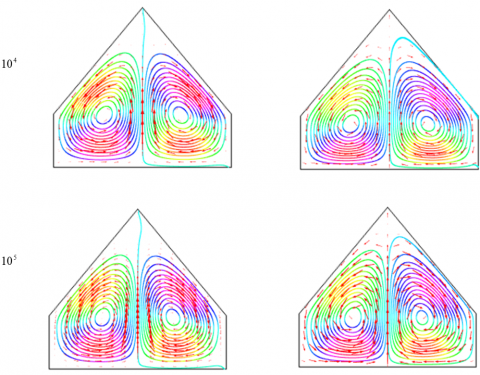## 3b.png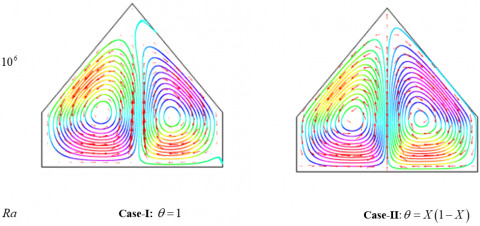Figure 3.  Streamlines for different values of Rayleigh number $\left( Ra \right)$ with two different thermal boundary conditions

## 4a.png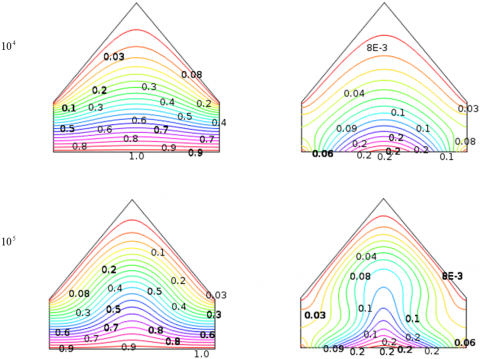## 4b.png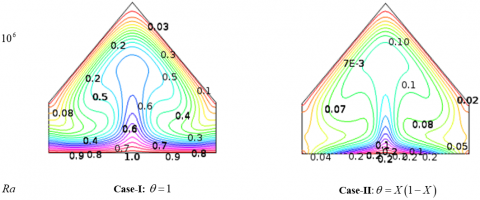Figure 4. Isotherms for different values of Rayleigh number $\left( Ra \right)$ with two different thermal boundary conditions

## 5a.png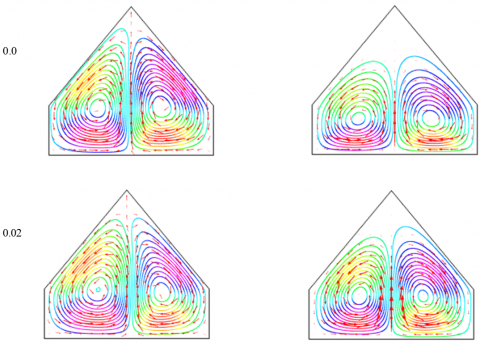## 5b.pngFigure 5. Streamlines for different values of nanoparticles volume fraction $\left( \phi \right)$ with two different thermal boundary conditions

## 6a.png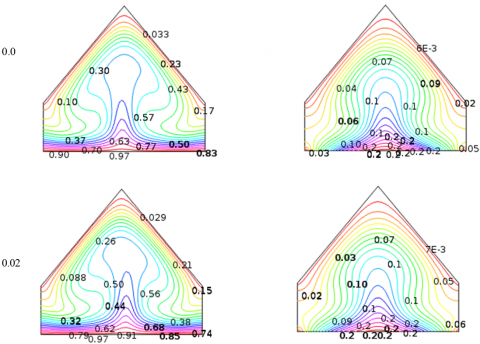## 6b.png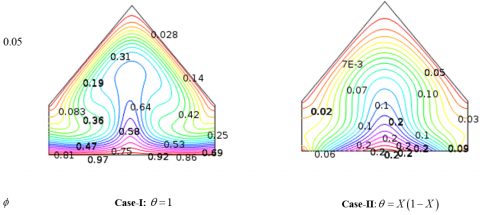Figure 6. Isotherms for different values of nanoparticles volume fraction $\left( \phi \right)$ with two different thermal boundary conditions

## 7.png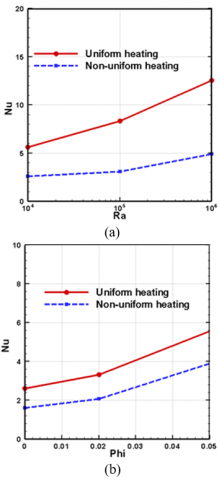Figure 7. Average Nusselt number for different values of (a) Rayleigh number $\left( Ra \right)$and (b) nanoparticles volume fraction $\left( \phi \right)$for both uniform and non-uniform heating bottom wall

11. Conclusions

The present investigation performed a physical as well as computational insight due to unsteady natural convective heat transfer and fluid flow of copper-water nanofluid with two different thermal boundary condition on the bottom wall. From the numerical computations, the following major conclusions may be drawn:

• Heat transfer rate increases with the increasing values of the nanoparticles volume fraction $\left( \phi \right)$for both cases.
• An increase in the Rayleigh number ($Ra$) is sufficient to increase the convective heat transfer effectively.
• For lower values of $Ra$,  conduction is the primary mode of heat transfer for all values of the other parameters.
• For higher value of Rayleigh number $(Ra)$, better heat transfer is observed  through convection and conduction.
• The highest heat transfer rate is obtained for uniformly bottom heated wall case.
Acknoeledgement

The author is grateful to The Research Council (TRC) of Oman  for a Postdoctoral Fellowship under the Open Research Grant Program: ORG/SQU/CBS/14/007.

Nomenclature
 $g$ acceleration due to gravity $L$ length of the base wall of the cavity $Nu$ Average Nusselt number $p$ dimensional fluid pressure $P$ dimensionless fluid pressure $\Pr$ Prandtl number $Ra$ Rayleigh number t dimensional time $T$ dimensional temperature $u,v$ dimensional velocity components $U,V$ dimensionless velocity components $W$ height of the vertical walls of the cavity $x,y$ dimensional coordinates $X,Y$ dimensionless coordinates $\alpha$ thermal diffusivity $\beta$ coefficient of thermal expansion $\upsilon$ kinematic viscosity $\rho$ density $\theta$ dimensionless temperature $\tau$ Dimensionless time Subscripts nf nanofluid bf base fluid sp solid particle h hot temperature c cold temperature
References

 Choi S., “Enhancing thermal conductivity of fluids with nanoparticles.” In: Signier DA, Wang HP (eds.) Development and applications of non-Newtonian flows, ASME FED, vol.231/MD, pp. 99–105, 1995.

 Choi S., “Nanofluid technology: current status and future research,” Energy Technology Division, Argonne National Laboratory, Argonne, 1999.

 Uddin M. J., Al Kalbani K. S., Rahman M. M., Alam M. S., Al-Salti N. and Eltayeb I. A., “Fundamentals of nanofluids: evolution, applications and new theory,” J. Biomath. Sys. Biolgy., vol. 1, no. 2, pp. 1-32, 2015.

 Parvin S.,  Chowdhury, R. , Khan, M. A. H. and Alim, M. A., “Performance of nanofluid in free convective heat transfer inside a cavity with non-isothermal boundary conditions,” Proceedings of the International Conference on Mechanical Energineering and renewable Energy, pp. 1-6, 26-29 November, 2015, Chittagong, Bangladesh.

 Zienkiewicz O. C. and Taylor R. L., “The finite element method,” 4th Edition, McGraw-Hill, 1991.

 Reddy, J. N. and Gartling, “The finite element method in heat transfer and fluid dynamics,” CRC Press, Boca Raton, Florida, 1994.

 Holtzman G. A., Hill R. W. and Ball K. S.,  “Laminar natural convection in isosceles triangular enclosures heated from below and symmetrically cooled from above,” ASME J. Heat Transfer, vol. 122, pp. 485- 491, 2000.